# Multiple If Statements in Excel (Nested Ifs, AND/OR) with Examples

If you want to test more than one condition then you need to use the If statement. The “If Statement” is used in many programming languages.

The Excel users also use this formula so much in their daily life to test conditions.

There are various ways to apply the If statement. Here you can check multiple if statements in excel using Nested Ifs and Logical test.

## Multiple If Statements in Excel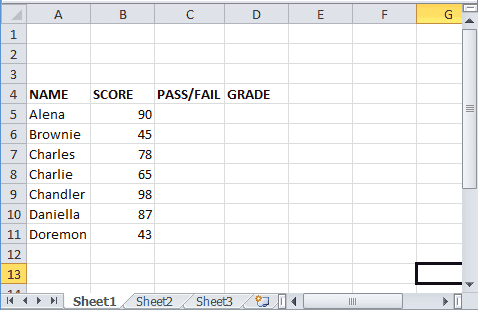In Excel, there are many ways to use If statements. Here we are going to describe all if the functions that one can use to test more than one condition.

### Excel If Statement

This is the simple or basic If statement which is used to test conditions that can return two results i.e, either TRUE or FALSE.

• Let’s take a data set which is shown above.
• Here we use Excel If contains data set to check whether the student is Pass or fail based on his or her scores.
• To perform this, we apply Excel if formula. The syntax of this formula is

=IF(test,[true],[false])

• As per the above data set, a student whose score is less than 60 is considered to fail.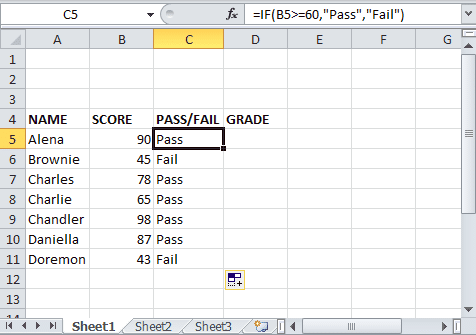• The formulas we apply in C5 is =IF(B5>=60,”Pass”,”Fail”).
• Check the result.

### Nested If

Here we are going to tell you how to use nested if. The term nesting means combining formulas together (one inside the other). By doing so, one formula will handle the outcome of another formula. When we talk of simple nested if, then it means one If Statement appears inside the other if statement.

• Take the above dataset.
• To perform Nested If, we need to use two if functions together. Here in first if we will check whether the student is pass or fail based on marks. In other, if function, we will check whether the content in the cell is empty or filled.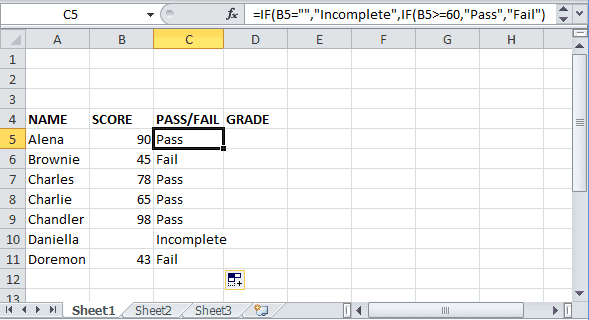• The formula we will put in C5 is =If(B5=””,”Incomplete”,If(B5>=60,”Pass”,”Fail”).
• Check the outcome.

### Excel If Statement Multiple Conditions Range

If you have too many conditions range then how to apply multiple if statements in excel? Here you can check the process.

• Here we are going to take the same data set.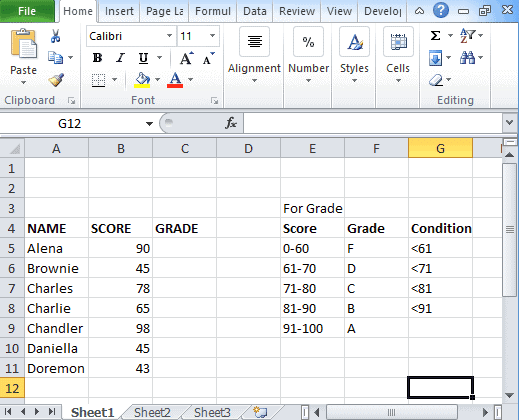• But the conditions to get an outcome are different.
• According to the conditions, the formula become

=IF(B5<61,”F”,IF(B5<71,”D”,IF(B5<81,”C”,IF(B5,”B”,”A”))))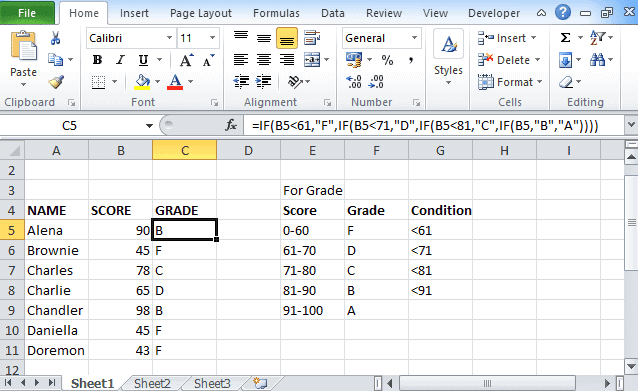• After putting this formula, check the outcome.

### Excel If Statement with Logical Test (AND/OR)

If you want to evaluate the sets of various conditions then you can use the OR/AND function. For this, you need to test the functions.

#### Excel If and function

If you want to test multiple conditions and want every condition evaluates to true, then you need to use the AND function.

• Take a dataset listed in the screenshot.
• To evaluate commission, put the formula in the Commission box.
`=IF(AND(B2>=150,C2>=150),10%,IF(AND(B2>=101,C2>=101),7%,IF(AND(B2>=51),5%,IF(AND(B2>=11,C2>=1),3%,""))))`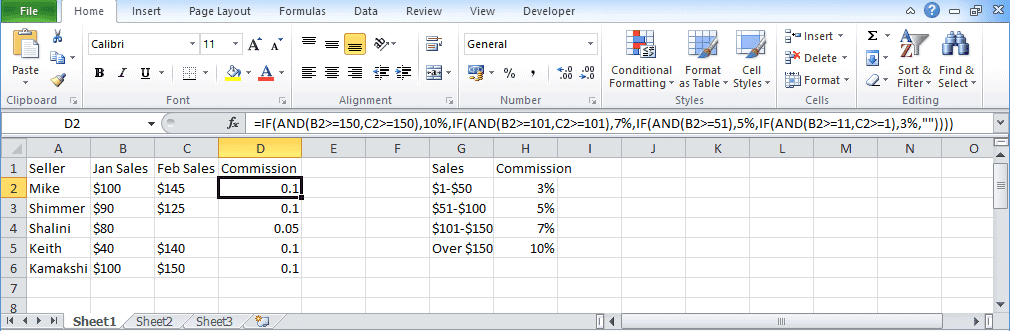• Check the outcome.

#### Excel If or function

Using this function, you can check two or more conditions using excel logical test and it should return TRUE if any of the condition evaluates to TRUE.

• Take the above dataset.
• Evaluate the commission by putting down the formula.
`=IF(OR(B2>=150,C2>=150),10%,IF(OR(B2>=101,C2>=101),7%,IF(OR(B2>=51,C2>=51),5%,IF(OR(B2>=11,C2>=1),3%,""))))`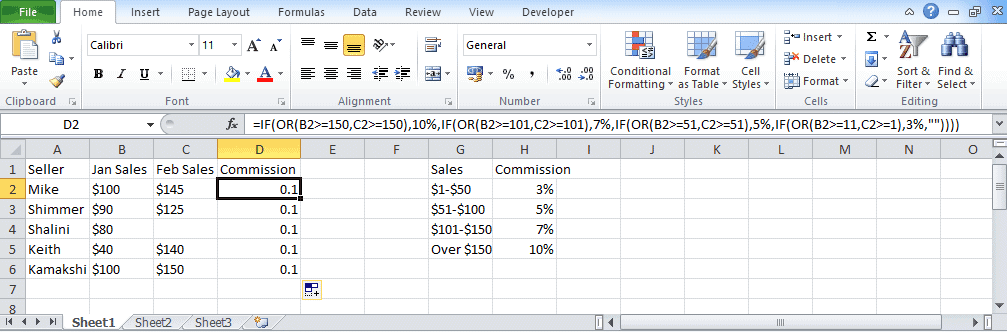• Check the result after putting down the formula.

So these are the different ways to use multiple if statements in excel. Try out all of these and check the result you get.

Other Excel tutorials you may find useful: